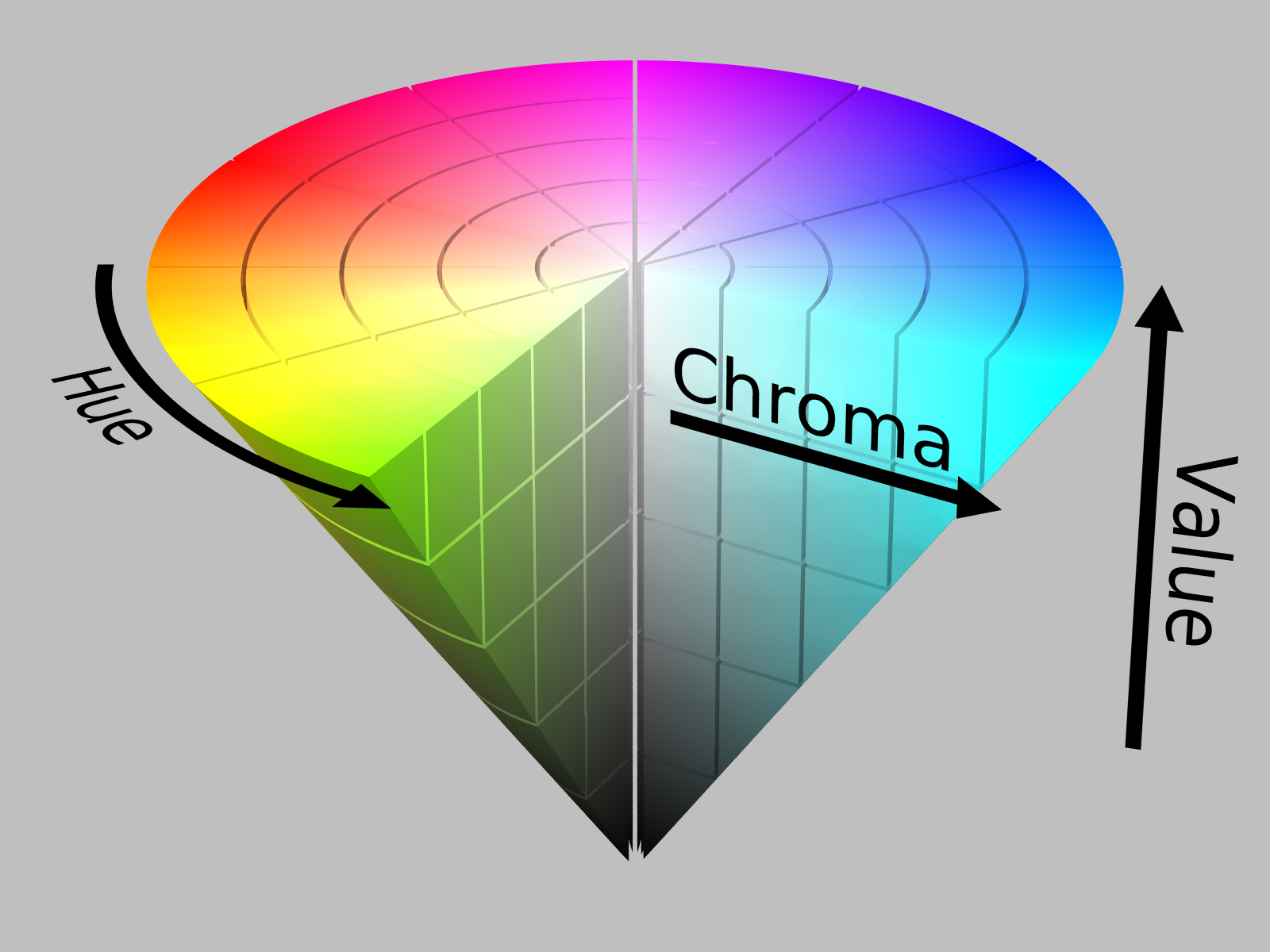# Basic Color Tracking with OpenCV

OpenCV is a fantastic library for use in everything computer vision. It has pretty good documentation and support, as well as being completely open source. Here’s an introduction to implementing a basic OpenCV program, which we’ll use to track a red circle.

A complete version of the code is at the end of this post.

```import cv2

def main():
cap = cv2.VideoCapture(0)
while True:
cv2.imshow("camera", frame)
c = cv2.waitKey(1)

if __name__ == '__main__':
main()
```

We open a connection to the webcam at index 0 (this number changes if you have multiple camera devices on your system) returning the image to the frame variable that we’ve set from before. We use `imshow` to show the frame to the screen, then pause for a second for the image to display. Run the code, and you should see a window with your webcam input pop up on the screen.Cool! So we have a frame for our OpenCV script to work with. Now, in image processing, we have to treat images as an array of numbers. In our case, the camera returns a 640×480 array of pixels with values from (0,0,0) to (255,255,255)–RGB color space. We’ll want to convert the RGB image into HSV for color filtering.

Typically, we visualize RGB(right) as a cube and HSV(left) as a cone.Note that RBG combines intensity and color. However, HSV separates the intensity of the color from the hue, which means we can simply filter one channel of the HSV image to get the color we want. Super useful!

**Note that OpenCV orders the channels as BRG instead of RGB**

Let’s convert the image to HSV, then filter for red.

```import cv2
import cv2.cv as cv
import numpy as np

def main():
cap = cv2.VideoCapture(0)
while True:

hsv_image = cv2.cvtColor(frame, cv2.COLOR_BGR2HSV)
red_image = cv2.inRange(hsv_image, (0,50,50), (8,170,200))
cv2.imshow("camera", frame)
cv2.imshow("red image", red_image)
c = cv2.waitKey(1)

if __name__ == '__main__':
main()
```Woah! So cool right!? Note that there’s a bit of red from my face, as well as my my little red friend in the background is highlighted as well. However, the majority of the filtered image is around the red pokeball. As a brief aside: The reason why color filtering isn’t the best way do object recognition is that color changes drastically with the lighting conditions. (Remember the blue/black, gold/white dress scandal earlier this year? Hahaha). To change the color that the code will filter for, change the value inside the cv2.inRange function.

With the red image filtered out now, we can do some basic shape detection to find where our ball is. The function can use for this is HoughCircles. The method behind circle detection is rather complex, but we can treat it like a black box for now and just run the code over our image.

```import cv2
import cv2.cv as cv
import numpy as np

def main():
cap = cv2.VideoCapture(0)
while True:

hsv_image = cv2.cvtColor(frame, cv2.COLOR_BGR2HSV)
red_image = cv2.inRange(hsv_image, (0,50,50), (8,170,200))

if circles != None:
circles = np.uint16(np.around(circles))
for i in circles[0,:]:
# draw the outer circle
cv2.circle(frame,(i,i),i,(0,255,0),2)
# draw the center of the circle
cv2.circle(frame,(i,i),2,(0,0,255),3)

cv2.imshow("camera", frame)
cv2.imshow("red image", red_image)
c = cv2.waitKey(1)

if __name__ == '__main__':
main()
```

The result is a function that can largely find red circular objects in a scene.A caveat:
This is a crazy basic implementation of color filtering + HoughCircles. As a result, this code only filters for a limited range of red colors, then runs the hough algorithm with fairly loose parameters over the resulting image. Due to this, this code is inflexible in different lightning conditions and is unreliable for the circle detected output. There are several ways to do object detection/color filtering, the intracacies of which I’ll cover another day. 🙂